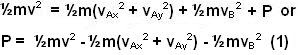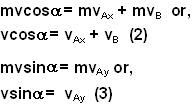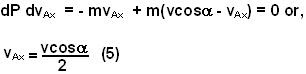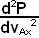## Monday, June 2, 2008

### Irodov Problem 1.170Lets label the striking ball as ball A and the stationary ball as ball B. Consider what happens during the small amount of time when the balls are in contact with each other during the collision. Ball A exerts a force R on ball B along the line joining their centers (since the balls contact point is on the line connecting their centers as shown in the figure). Ball B then exerts an equal and opposite force R on ball A. Let us label the line joining the centers of balls A and B at the time of contact as the x-axis.

The force R acting on ball B, accelerates the ball along the x-axis and finally when the balls separate ball B will be moving along the x-axis.

The force R acting on ball A, acts along the x-axis and thus will reduce the x component of ball A's velocity. In other words R will i) rotate the velocity vector (change the direction of motion) of ball A, and ii) decrease the magnitude (slow) balls A. This is depicted in the figure.

Consider some instant of time during the collision (when balls are still in contact not yet separated). Suppose that this point, ball B has acquired a velocity vB velocity along the x direction and ball A has a velocity (vAx i + vAy j) velocity. The net kinetic energy of the system is given by. The initial kinetic energy of the system was simply. Let the potential energy of compression during the collision at this instant be P. Since the total energy must always be conserved we have,Note that this is only during the time the balls are in contact, when the balls separate there will be no potential energy of compression and P=0.

Since there are no external forces acting on the system, the momentum will also always be conserved at any instant of time (both during the balls are in contact or not). Conserving momenta in x and y directions we have,From (2) and (3) we have,The compression potential energy will be maximized when,It is easy to see that the second derivativeat this value will be -2m which is negative.

Substituting this in (2) and (4) we have,The fraction of the initial energy that is stored as compression potential is thus given by.